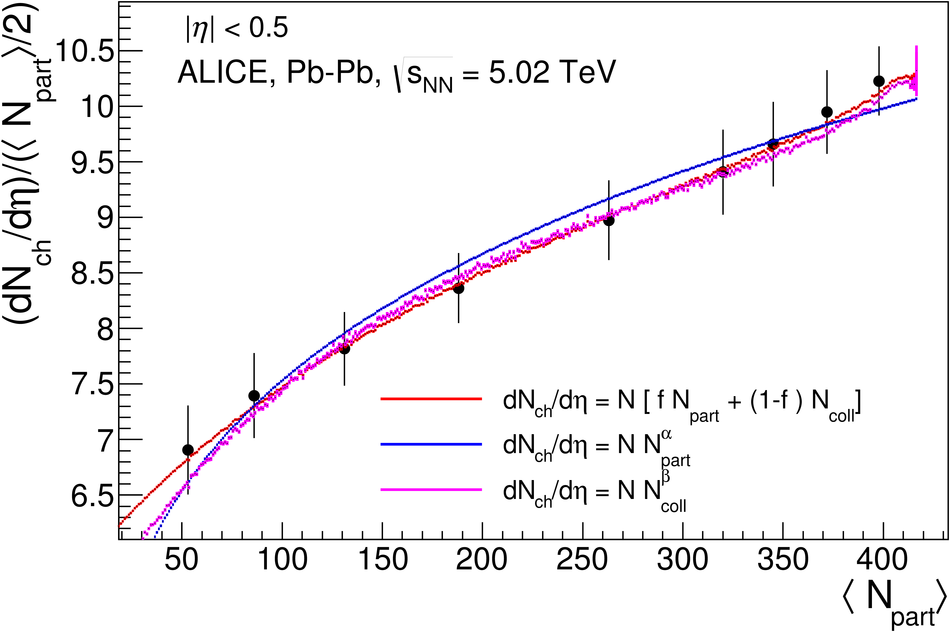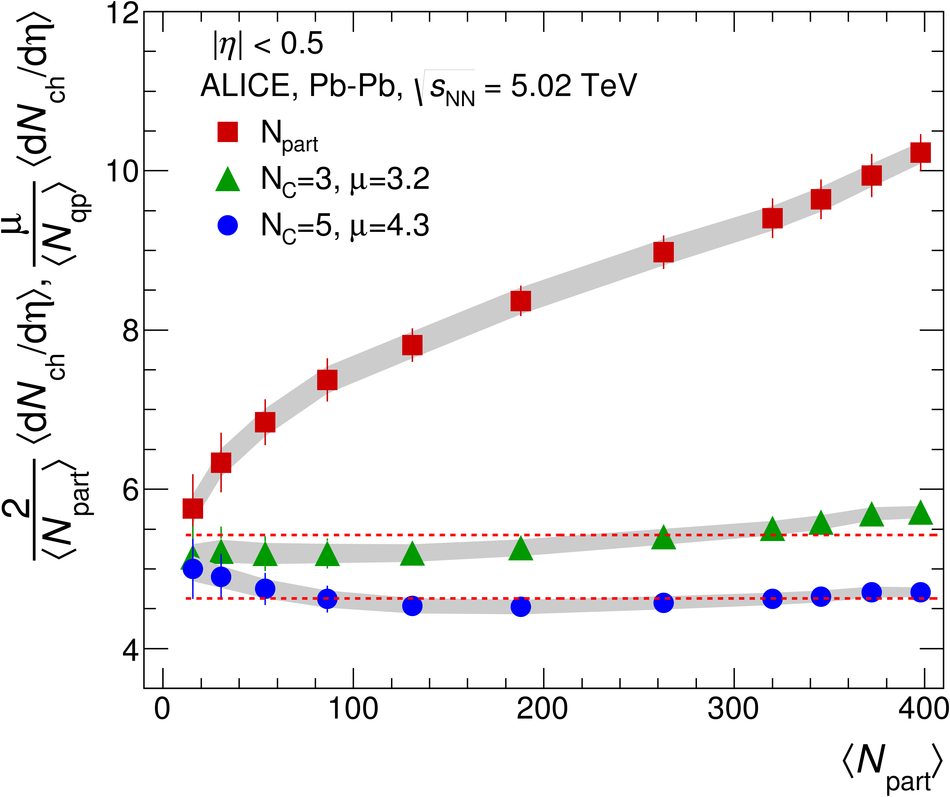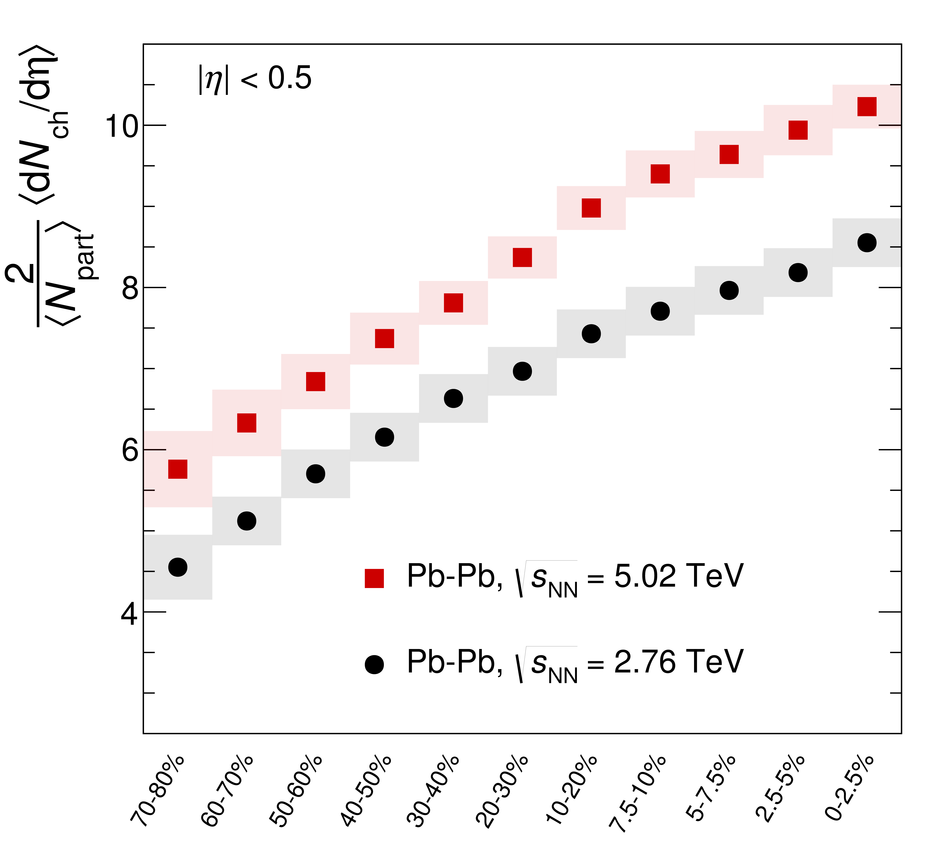# Centrality dependence of the charged-particle multiplicity density at mid-rapidity in Pb-Pb collisions at $\sqrt{s_{\rm NN}}$ = 5.02 TeV

The pseudorapidity density of charged particles ($\mathrm{d}N_\mathrm{ch}/\mathrm{d}\eta$) at mid-rapidity in Pb-Pb collisions has been measured at a center-of-mass energy per nucleon pair of $\sqrt{s_{\rm NN}}$ = 5.02 TeV. It increases with centrality and reaches a value of $1943 \pm 54$ in $|\eta|<~0.5$ for the 5% most central collisions. A rise in $\mathrm{d}N_\mathrm{ch}/\mathrm{d}\eta$ as a function of $\sqrt{s_{\rm NN}}$ for the most central collisions is observed, steeper than that observed in proton-proton collisions and following the trend established by measurements at lower energy. The centrality dependence of $\mathrm{d}N_\mathrm{ch}/\mathrm{d}\eta$ as a function of the average number of participant nucleons, ${\langle N_\mathrm{part} \rangle}$, calculated in a Glauber model, is compared with the previous measurement at lower energy. A constant factor of about 1.2 describes the increase in $\frac{2}{\langle N_\mathrm{part} \rangle}\langle \mathrm{d}N_\mathrm{ch}/\mathrm{d}\eta \rangle$ from $\sqrt{s_{\rm NN}}$ = 2.76 TeV to $\sqrt{s_{\rm NN}}$ = 5.02 TeV for all centrality intervals, within the measured range of 0-80% centrality. The results are also compared to models based on different mechanisms for particle production in nuclear collisions.

Figures

## Figure 1

 Values of $\frac{2}{\langle N_{\rm part}\rangle}\langle$d$N_{\rm ch}$d$\eta\rangle$ for central Pb-Pb and Au-Au collisions (see text) as a function of $\snn$. Measurements for inelastic pp and ${\rm p}\bar{\rm p}$ collisions as a function of $\sqrt{s}$ are also shown along with those from non-single diffractive p-A and d-A collisions. The $s$-dependencies of the AA and pp (${\rm p}\bar{\rm p}$) collision data are well described by the functions $s_{\rm NN}^{0.155}$ (solid line) and $s_{\rm NN}^{0.103}$ (dashed line), respectively The shaded bands show the uncertainties on the extracted power-law dependencies. The central Pb-Pb measurements from CMS and ATLAS at 2.76 TeV have been shifted horizontally for clarity.## Figure 2

 The $\frac{2}{\langle N_{\rm part}\rangle}\langle$d$N_{\rm ch}$d$\eta\rangle$ for Pb-Pb collisions at $\snn$ = 5.02 TeV in the centrality range 0-80$\%$, as a function of $\langle N_{\mathrm{part}}\rangle$ in each centrality class. The error bars indicate the point-to-point centrality-dependent uncertainties whereas the shaded band shows the correlated contributions. Also shown is the result from non-single diffractive p-Pb collisions at the same $\snn$. Data from lower energy (2.76 TeV) Pb-Pb and pp collisions, scaled by a factor of 1.2 and 1.13 respectively, are shown for comparison. The error bars for p-Pb at $\snn$ = 5.02 TeV and lower energy Pb-Pb and pp collisions indicate the total uncertainty.## Figure 3

 The $\frac{2}{\langle N_{\rm part}\rangle}\langle$d$N_{\rm ch}$d$\eta\rangle$ for Pb-Pb collisions at $\snn$ = 5.02 TeV in the centrality range 0-80$\%$, as function a of $\langle N_{\mathrm{part}} \rangle$ in each centrality class, compared to model predictions .## Figure 1

 Distribution of the sum of amplitudes in the V0 scintillators. The distribution is fitted with the NBD-Glauber fit (explained in the text) shown as a line. The insert shows a zoom of the most peripheral region.## Figure 2

 Centrality dependence of $\dNdeta$ per participant pair as a function of $\Npart$, measured in the Pb-Pb data at $\snn = 5.02$ TeV fitted with various parameterizations of $\Npart$ and $\Ncoll$, calculated with the Glauber model. Data are from .## Figure 3

 Centrality dependence of $\dNdeta$ per participant nucleon, $\Npart$, calculated with the single-quark scattering model, as a function of $\Npart$, measured in the Pb-Pb data at $\snn = 5.02$ TeV. The $\dNdeta$ data are also divided by $\Npart$, calculated from the standard Glauber MC. The dashed lines indicate a fit with a constant.## Figure 4

 $\dNdeta$ as a function of the centrality classes at $\snn$ = 2.76 and 5.02 TeV## Figure 5

 Ratio of $\dNdeta$ per participant pairs at $\snn$ = 2.76 and 5.02 TeV, as a function of the centrality classes. The data has been normalized to $\Npart$ calculated from a Glauber Monte Carlo.